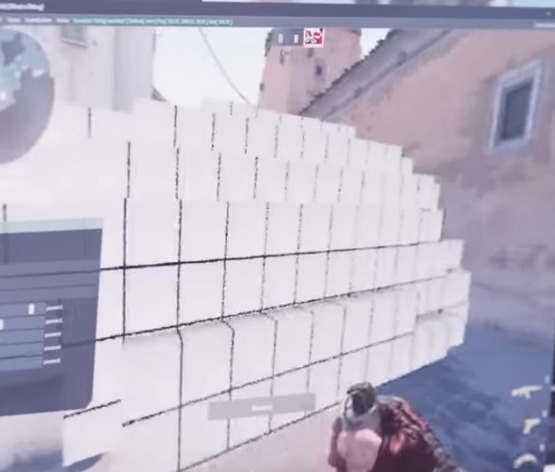# How do I create a sphere made of multiple parts?

So, I’m trying to make a sphere made of multiple cubes, something like this:Yes, I’m trying to make cs2 smoke in roblox, but I just need a way to make a sphere out of cubes like this. How would I do this? I need to be able to control the cube size and sphere size but I have no idea how.

``````local diameter = 10
local chunk = Vector3.one * 5 -- vector3.new(5,5,5) -- the blocky blocks size
local pos = Vector3.zero -- vector3.new(0,0,0)
local r = diameter/2
local t = (chunk.X + chunk.Y + chunk.Z)/3
for x = -r, r, t do
for y = -r, r, t do
for z = -r, r, t do
local pos2 = pos + Vector3.new(x, y, z)
if ((pos2 - pos).Magnitude > (r)) then return end;
local part = Instance.new("Part", workspace) -- parented on workspace
part.Size = chunk
part.CFrame = CFrame.new(pos2)
part.Anchored = true
end
end
end
``````

idk if it works, save your game first before your computer crashes (maybe)

This topic was automatically closed 14 days after the last reply. New replies are no longer allowed.